Simplify data visualization in Python with Plotly

Plotly is a data plotting library with a clean interface designed to allow you to build your own APIs.Image by :
Pixabay. Modified by Opensource.com. CC BY-SA 4.0.

Subscribe now

Get the highlights in your inbox every week.

Plotly is a plotting ecosystem that allows you to make plots in Python, as well as JavaScript and R. In this series of articles, I'm focusing on plotting with Python libraries.

Plotly has three different Python APIs, giving you a choice of how to drive it:

I'll explore each of these APIs by making the same plot in each one: a grouped bar plot of historical UK election results.

Before we go further, note that you may need to tune your Python environment to get this code to run, including the following.

• Running a recent version of Python (instructions for LinuxMac, and Windows)
• Verify you're running a version of Python that works with these libraries

The data is available online and can be imported using pandas:

import pandas as pd

Making plots using Graph Objects

Plotly's object-oriented API is named graph_objects. It's somewhat similar to Matplotlib's object-oriented API.

To create a multi-bar plot, you can construct a figure object containing four bar plots:

# Import Plotly and our data
import plotly.graph_objects as go

# Get a convenient list of x-values
years = df['year']
x = list(range(len(years)))

# Specify the plots
bar_plots = [
go.Bar(x=x, y=df['conservative'], name='Conservative', marker=go.bar.Marker(color='#0343df')),
go.Bar(x=x, y=df['labour'], name='Labour', marker=go.bar.Marker(color='#e50000')),
go.Bar(x=x, y=df['liberal'], name='Liberal', marker=go.bar.Marker(color='#ffff14')),
go.Bar(x=x, y=df['others'], name='Others', marker=go.bar.Marker(color='#929591')),
]

# Specify the layout
layout = go.Layout(
title=go.layout.Title(text="Election results", x=0.5),
yaxis_title="Seats",
xaxis_tickmode="array",
xaxis_tickvals=list(range(27)),
xaxis_ticktext=tuple(df['year'].values),
)

# Make the multi-bar plot
fig = go.Figure(data=bar_plots, layout=layout)

# Tell Plotly to render it
fig.show()

Unlike in Matplotlib, there's no need to calculate the x-positions of the bars manually; Plotly takes care of that for you.

Here's the final plot:

plotly.png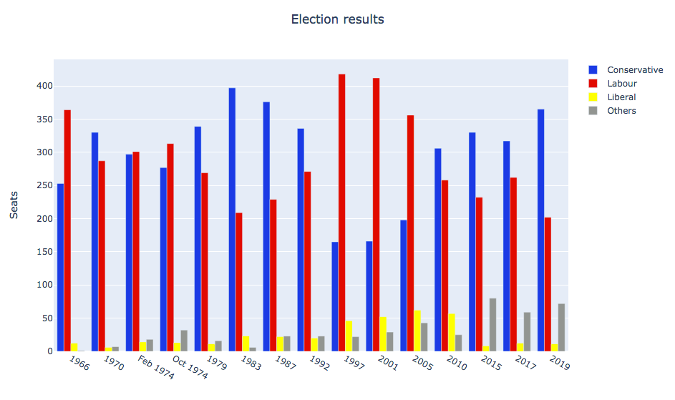Making plots using Python data structures

You can also specify your plot using basic Python data structures with the same structure as the object-oriented API. This corresponds directly to the JSON API for Plotly's JavaScript implementation.

# Specify the plots
fig = {
'data': [
{'type': 'bar', 'x': x, 'y': df['conservative'], 'name': 'Conservative', 'marker': {'color': '#0343df'}},
{'type': 'bar', 'x': x, 'y': df['labour'], 'name': 'Labour', 'marker': {'color': '#e50000'}},
{'type': 'bar', 'x': x, 'y': df['liberal'], 'name': 'Liberal', 'marker': {'color': '#ffff14'}},
{'type': 'bar', 'x': x, 'y': df['others'], 'name': 'Others', 'marker': {'color': '#929591'}},
],
'layout': {
'title': {'text': 'Election results', 'x': 0.5},
'yaxis': {'title': 'Seats'},
'xaxis': {
'tickmode': 'array',
'tickvals': list(range(27)),
'ticktext': tuple(df['year'].values),
}
}
}

# Tell Plotly to render it
pio.show(fig)

The final plot looks exactly the same as the previous plot:

plotly-pio.png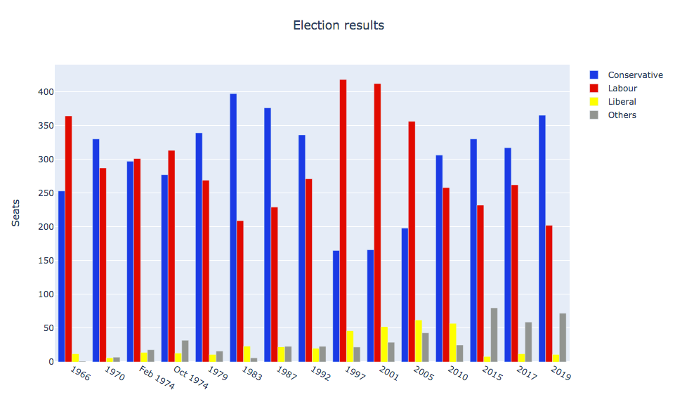Making plots using Plotly Express

Plotly Express is a high-level API that wraps Graph Objects.

You can make a multi-bar plot in Plotly Express using (almost) a single line:

# Import Plotly and our data
import plotly.express as px

# Define the colourmap to get custom bar colours
cmap = {
'Conservative': '#0343df',
'Labour': '#e50000',
'Liberal': '#ffff14',
'Others': '#929591',
}

# Make the plot!
fig = px.bar(df, x="year", y="seats", color="party", barmode="group", color_discrete_map=cmap)

This makes use of the data in Long Form, also known as "tidy data." The columns are year, party, and seats, rather than being split by party. It's very similar to making a multi-bar plot in Seaborn.

>> print(long)
year         party  seats
0    1922  Conservative    344
1    1923  Conservative    258
2    1924  Conservative    412
3    1929  Conservative    260
4    1931  Conservative    470
..    ...           ...    ...
103  2005        Others     30
104  2010        Others     29
105  2015        Others     80
106  2017        Others     59
107  2019        Others     72

[108 rows x 3 columns]

You can access the underlying Graph Objects API to make detailed tweaks. Add a title and a y-axis label:

# Use the Graph Objects API to tweak our plot
import plotly.graph_objects as go
fig.layout = go.Layout(
title=go.layout.Title(text="Election results", x=0.5),
yaxis_title="Seats",
)

And finally, ask Plotly to show it to you:

# Tell Plotly to render it
fig.show()

This runs a temporary web server on an unused port and opens the default web browser to view the plot (the webserver is immediately torn down).

Unfortunately, the result is not perfect. The x-axis is treated as an integer, so the groups are far apart and small. This makes it quite difficult to see trends.

plotly-express.png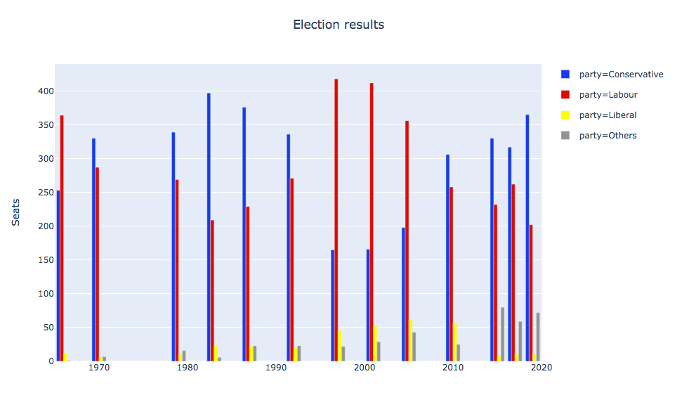You might try to encourage Plotly Express to treat the x-values as strings by casting them to strings. You might expect this to result in them being plotted with even spacing and lexical ordering. Unfortunately, you still get them helpfully spaced numerically. Setting the xaxis_tickvals does not work as it did in graph_objects, either.

Unlike the similar example in Seaborn, in this case, the abstraction does not appear to provide sufficient escape hatches to provide things exactly how you want them. But perhaps you could write your own API?

Not happy with how Plotly does something? Build your own Plotly API!

At its core, Plotly is a JavaScript library that makes plots using D3 and stack.gl. The JavaScript library has an interface that consumes JSON structures that specify plots. So you just need to output JSON structures that the JavaScript library likes to consume.

Anvil did that to create a Python Plotly API that works entirely in the browser.

plotly-arch.png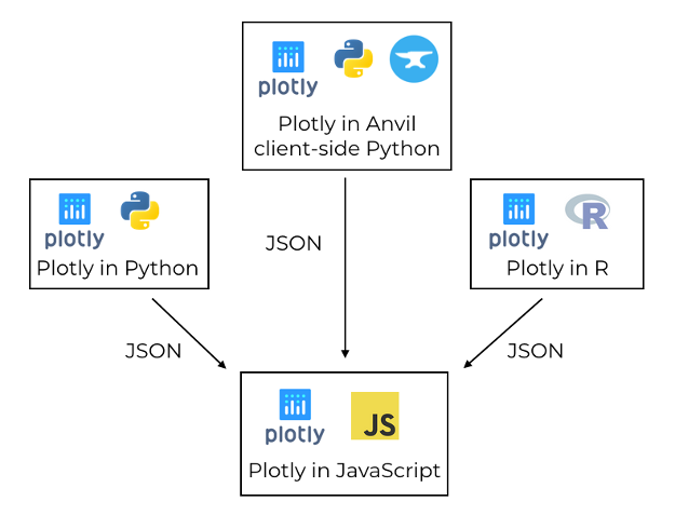Plotly uses a JavaScript library to create plots, driven by libraries in other languages via JSON (© 2019 Anvil)

In the Anvil version, you can use both the Graph Objects API and the Python data structure approach explained above. You run exactly the same commands, assigning the data and layout to a Plot component in your Anvil app.

Here's the multi-bar plot written in Anvil's client-side Python API:

# Import Anvil libraries
from ._anvil_designer import EntrypointTemplate
from anvil import *
import anvil.server

# Import client-side Plotly
import plotly.graph_objs as go

# This is an Anvil Form
class Entrypoint(EntrypointTemplate):
def __init__(self, **properties):
# Set Form properties and Data Bindings.
self.init_components(**properties)

# Fetch the data from the server
data = anvil.server.call('get_election_data')

#  Get a convenient list of x-values
years = data['year']
x = list(range(len(years)))

# Specify the plots
bar_plots = [
go.Bar(x=x, y=data['conservative'], name='Conservative', marker=go.Marker(color='#0343df')),
go.Bar(x=x, y=data['labour'], name='Labour', marker=go.Marker(color='#e50000')),
go.Bar(x=x, y=data['liberal'], name='Liberal', marker=go.Marker(color='#ffff14')),
go.Bar(x=x, y=data['others'], name='Others', marker=go.Marker(color='#929591')),
]
# Specify the layout
layout = {
'title': 'Election results',
'yaxis': {'title': 'Seats'},
'xaxis': {
'tickmode': 'array',
'tickvals': list(range(27)),
'ticktext': data['year'],
},
}

# Make the multi-bar plot
self.plot_1.data = bar_plots
self.plot_1.layout = layout

The plotting logic is the same as above, but it's running entirely in the web browser—the plot is created by the Plotly JavaScript library on the user's machine! This is a big advantage over all the other Python plotting libraries in this series. All the other Python libraries need to run on a server.

Here's the interactive Plotly plot running in an Anvil app:

plotting-in-anvil.gif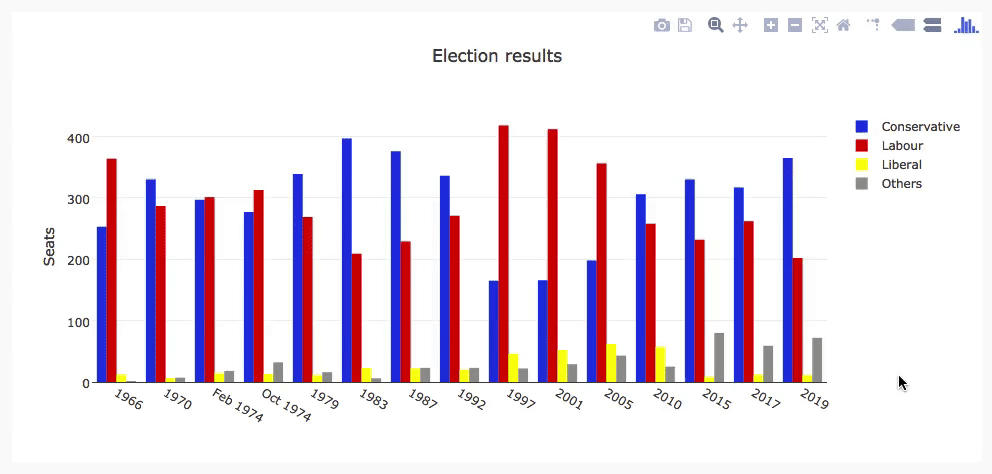The election plot on the web using Anvil's client-side-Python Plotly library (© 2019 Anvil)

You can copy this example as an Anvil app (Note: Anvil requires registration to use).

Running Plotly in the frontend has another advantage: it opens up many more options for customizing interactive behavior.

Customizing interactivity in Plotly

Plotly plots aren't just dynamic; you can customize their interactive behavior. For example, you can customize the format of tool-tips using hovertemplate in each bar plot:

go.Bar(
x=x,
y=df['others'],
name='others',
marker=go.bar.Marker(color='#929591'),
hovertemplate='Seats: <b>%{y}</b>',
),

Here's what you get when you apply this to each bar plot:

plotly-tooltips.png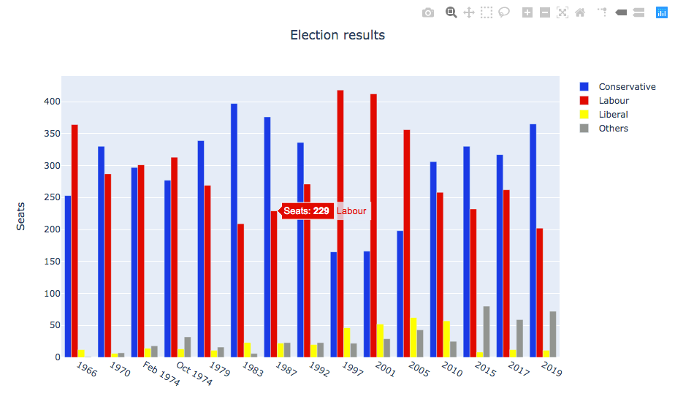A multi-bar plot with custom tool-tips (© 2019 Anvil)

This is useful, but it would be even better if you could execute any code you want when certain events happen—like when a user hovers over the bar and you want to display an information box about the relevant election. In Anvil's Plotly library, you can bind event handlers to events such as hover, which makes that sort of complex interactivity possible!

plotly-event-handling.gif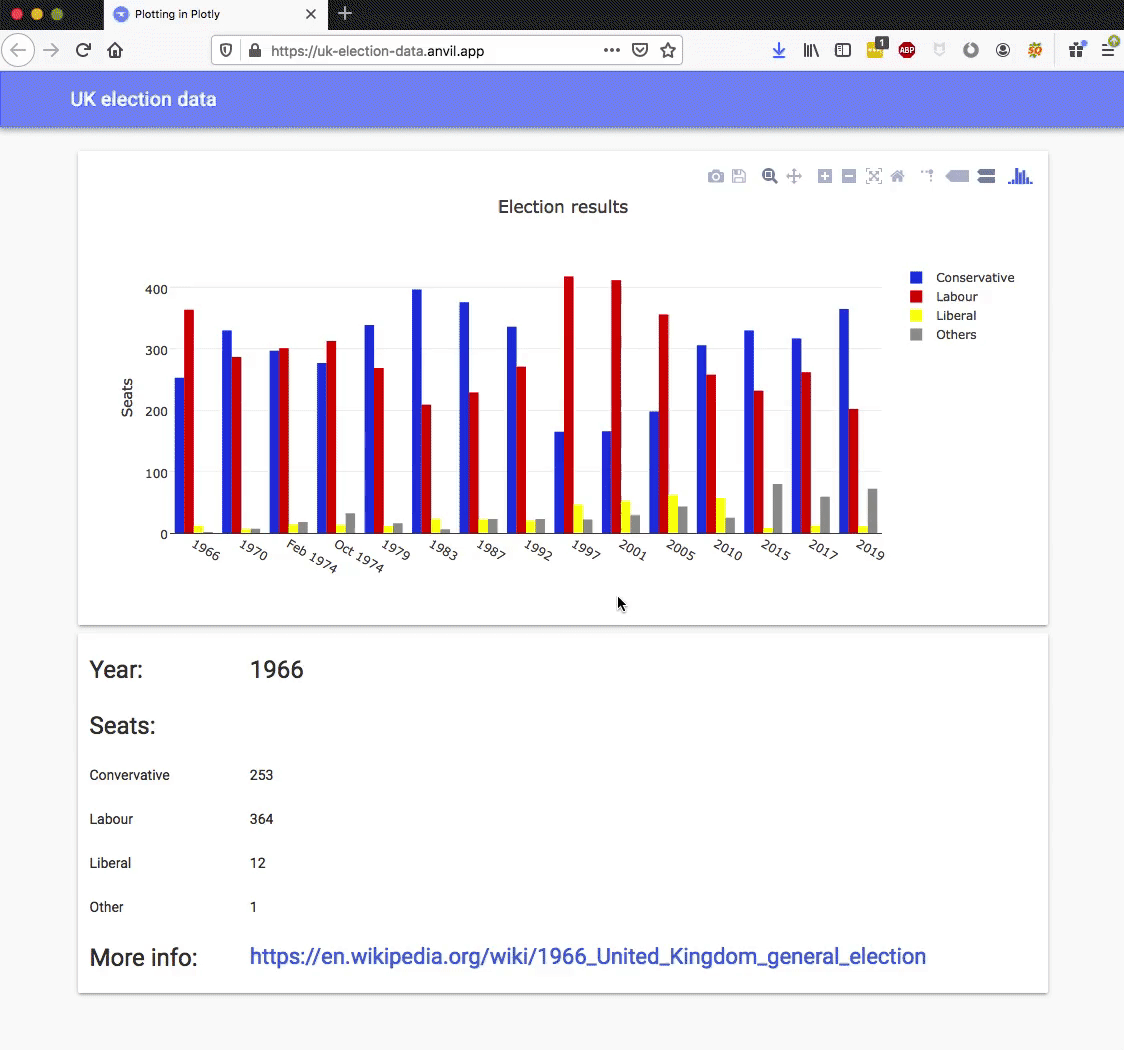A multi-bar plot with a hover event handler (© 2019 Anvil)

You can achieve this by binding a method to the plot's hover event:

def plot_1_hover(self, points, **event_args):
"""This method is called when a data point is hovered."""
i = points['point_number']
self.label_year.text = self.data['year'][i]
self.label_con.text = self.data['conservative'][i]
self.label_lab.text = self.data['labour'][i]
self.label_lib.text = self.data['liberal'][i]
self.label_oth.text = self.data['others'][i]
url = f"https://en.wikipedia.org/wiki/{self.data['year'][i]}_United_Kingdom_general_election"

This is a rather extreme level of interactivity, and from the developer's point of view, an extreme level of customizability. It's all thanks to Plotly's architecture—Plotly has a clean interface that is explicitly designed to allow you to build your own APIs. It would be helpful to see this kind of great design everywhere!

Custom interactivity using Bokeh

You've seen how Plotly uses JavaScript to create dynamic plots, and you can edit them live in the browser using Anvil's client-side Python code.

Bokeh is another Python plotting library that outputs an HTML document you can embed in a web app and get similar dynamic features to those provided by Plotly. (That's "BOE-kay," if you're wondering how to pronounce it.)

Enjoy customizing charts and share tips and tricks in the comments below.